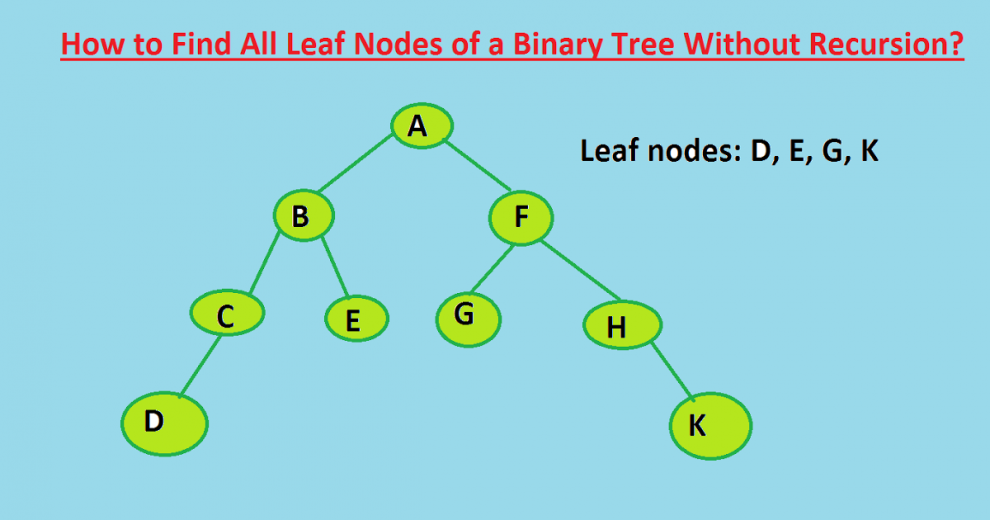# C Program – Inorder Tree Traversal without recursion and without stack!Inorder Tree Traversal without recursion and without stack! - Using Morris Traversal, we can traverse the tree without using stack and recursion.

Using Morris Traversal, we can traverse the tree without using stack and recursion. The idea of Morris Traversal is based on Threaded Binary Tree. In this traversal, we first create links to Inorder successor and print the data using these links, and finally revert the changes to restore original tree.

```1. Initialize current as root
2. While current is not NULL
If current does not have left child
a) Print current’s data
b) Go to the right, i.e., current = current->right
Else
a) Make current as right child of the rightmost
node in current's left subtree
b) Go to this left child, i.e., current = current->left
```

Although the tree is modified through the traversal, it is reverted back to its original shape after the completion. Unlike Stack based traversal, no extra space is required for this traversal.

``````#include<stdio.h>
#include<stdlib.h>

/* A binary tree tNode has data, pointer to left child
and a pointer to right child */
struct tNode
{
int data;
struct tNode* left;
struct tNode* right;
};

/* Function to traverse binary tree without recursion and
without stack */
void MorrisTraversal(struct tNode *root)
{
struct tNode *current,*pre;

if(root == NULL)
return;

current = root;
while(current != NULL)
{
if(current->left == NULL)
{
printf("%d ", current->data);
current = current->right;
}
else
{
/* Find the inorder predecessor of current */
pre = current->left;
while(pre->right != NULL && pre->right != current)
pre = pre->right;

/* Make current as right child of its inorder predecessor */
if(pre->right == NULL)
{
pre->right = current;
current = current->left;
}

/* Revert the changes made in if part to restore the original
tree i.e., fix the right child of predecssor */
else
{
pre->right = NULL;
printf("%d ",current->data);
current = current->right;
} /* End of if condition pre->right == NULL */
} /* End of if condition current->left == NULL*/
} /* End of while */
}

/* UTILITY FUNCTIONS */
/* Helper function that allocates a new tNode with the
given data and NULL left and right pointers. */
struct tNode* newtNode(int data)
{
struct tNode* tNode = (struct tNode*)
malloc(sizeof(struct tNode));
tNode->data = data;
tNode->left = NULL;
tNode->right = NULL;

return(tNode);
}

/* Driver program to test above functions*/
int main()
{

/* Constructed binary tree is
1
/   \
2      3
/  \
4     5
*/
struct tNode *root = newtNode(1);
root->left        = newtNode(2);
root->right       = newtNode(3);
root->left->left  = newtNode(4);
root->left->right = newtNode(5);

MorrisTraversal(root);

getchar();
return 0;
}``````

Output:

`4 2 5 1 3`
READ  C Algorithm - Iterative Postorder Traversal | Set 2 (Using One Stack)#### Venkatesan Prabu

Wikitechy Founder, Author, International Speaker, and Job Consultant. My role as the CEO of Wikitechy, I help businesses build their next generation digital platforms and help with their product innovation and growth strategy. I'm a frequent speaker at tech conferences and events.

X